Related Articles
Enhanced Binary Exponential Backoff Algorithm for Fair Channel Access in Ad Hoc Networks
• Difficulty Level : Hard
• Last Updated : 26 Mar, 2021

The Binary Exponential Backoff Algorithm prevents packet collisions during simultaneous access by randomizing moments at stations attempting to access the wireless channels. However, this randomization does not completely eliminate packet collisions, leading to reduced system throughput and increased packet delay and drop. Also, the BEB Algorithm results in unfair channel access among stations.

A mobile Ad hoc network is a group of wireless nodes that can dynamically arrange themselves into an arbitrary and temporary topology without the need for a pre-existing infrastructure to form a network. The node may move dynamically while communicating and makes the node incalculable.  The node can leave the topology network briskly.

Usually, this network is used in applications such as Emergency Operations, Environmental Control, and Military Applications where there is no centralized network infrastructure management or support, such as routers or Base Stations In the IEEE 802.11 standard, the distributed coordination function (DCF) algorithm is an efficient and fundamental algorithm that ensures the best MANET compatibility with evolving topology challenges. Based on the carrier sense multiple access with collision avoidance (CSMA/CA) protocol, the DCF shares access to the medium, which follows the basic principle of “listening before talking”. The BEB Algorithm is employed in DCF. After the collision, the node wishing to retransmit waits for a random amount of time which is known as the Backoff Time. Here, the node having small BT will first access the medium relative to the node with the higher BT.

Distributed Coordination Function:

This function is used to prevent collision using CSMA/CA and RTS/CTS acknowledgment frames. At the time of transmission, the sender waits for DIFS (Distributed Coordination Function Interframe Spacing) time. If the channel is idle it sends the data frame i.e RTS ACK frame while the receiver waits for the SIFS (Short Inter-Frame Spacing) amount of time and then sends the CTS frame receipt to the sender. If the channel is busy, the station waits for the channel until it is sensed idle for a DIFS time. At this point, it generates random BT to send the data frame.

### Binary Exponential Backoff Algorithm:

Upon successful data transmission, the function CW() is invoked to adjust the CW to CWmin. During data collisions, the node invokes the function Double CW() to double the CW until it is equal to or greater than CWmax.

If the node attempts to send data unsuccessfully seven times, the function CW() is also invoked to adjust CW to CWmin due to which packet delay and drop increases also reduce the probability of retransmission of the data frames. Also, the fairness problem occurs.

Fairness Problem:

If the Backoff time of a supposed node A is low as compared to node B then node A will reach zero first and reset the contention window to a minimum, due to which the first node transmits having a higher probability of transmitting its node again and again.

Consider a file “aaa.txt”. In this file, generate the 0s and 1s. Here, 0s mean unsuccessful, and 1s mean successful. Below is the text file:

1010011000100011

Below is the implementation of the above approach:

## C

 `// C program for the above approach``#include ``#include ``#include ``#include `` ` `// Driver Code``int` `main()``{``    ``// Slot time``    ``float` `slot_time = 0.00000166;`` ` `    ``int` `CWmin = 32, CWmax = 1024;`` ` `    ``int` `k;``    ``int` `i, Curr_BW = 32, counter = 0;``    ``float` `BT, Total_Backoff_time = 0;`` ` `    ``FILE``* fp;``    ``char``* f_name = ``"aaa.txt"``;``    ``char` `ch;``    ``int` `x = 0, y = 0;`` ` `    ``fp = ``fopen``(f_name, ``"r+"``);`` ` `    ``// Open File``    ``if` `(fp == NULL) {``        ``printf``(``"%s does not exists"``               ``" \n"``,``               ``f_name);``        ``return` `0;``    ``}`` ` `    ``// Otherwise``    ``else` `{``        ``printf``(``"%s: opened in read"``               ``" mode.\n\n"``,``               ``f_name);``    ``}`` ` `    ``// Read characters from the file``    ``while` `((ch = ``fgetc``(fp)) != EOF) {`` ` `        ``// End-of-file is reached``        ``if` `(ch == ``'1'` `|| ch == ``'0'``) {``            ``printf``(``"frame %c \n "``, ch);``        ``}`` ` `        ``// If the character is 1``        ``if` `(ch == ``'1'``) {`` ` `            ``x = x + 1;``            ``printf``(``"successful "``                   ``"Transmission\n"``);``            ``Curr_BW = CWmin;``            ``printf``(``"the current "``                   ``"window is : %d\n"``,``                   ``Curr_BW);`` ` `            ``BT = Curr_BW * slot_time;``            ``printf``(``" =>the backoff"``                   ``" time is : %0.8f"``                   ``" \n"``,``                   ``BT);`` ` `            ``counter = 0;``        ``}`` ` `        ``// If the character is 0``        ``else` `if` `(ch == ``'0'``) {`` ` `            ``y = y + 1;``            ``if` `(counter < 7) {`` ` `                ``printf``(``"UNsuccessful "``                       ``"Transmission\n"``);`` ` `                ``Curr_BW = Curr_BW * 2;`` ` `                ``if` `(Curr_BW > CWmax) {`` ` `                    ``Curr_BW = CWmax;``                ``}``                ``printf``(``"the current "``                       ``"window is :"``                       ``" %d\n"``,``                       ``Curr_BW);`` ` `                ``counter = counter + 1;``                ``printf``(``"counter is  :"``                       ``" %d \n "``,``                       ``counter);`` ` `                ``BT = Curr_BW * slot_time;`` ` `                ``printf``(``" =>the backoff"``                       ``" time is : %0.8f"``                       ``" \n"``,``                       ``BT);``            ``}`` ` `            ``// Otherwise``            ``else` `{`` ` `                ``Curr_BW = CWmin;``                ``printf``(``"the current"``                       ``" window is :"``                       ``" %d\n"``,``                       ``Curr_BW);`` ` `                ``BT = Curr_BW * slot_time;``                ``printf``(``" =>the backoff"``                       ``" time is : %0.8f"``                       ``" \n"``,``                       ``BT);``            ``}``        ``}`` ` `        ``if` `(ch == ``'1'` `|| ch == ``'0'``) {`` ` `            ``// Find the Backoff Time``            ``Total_Backoff_time = Total_Backoff_time + BT;`` ` `            ``printf``(``"\n"``);`` ` `            ``// Print the time``            ``printf``(``"=>  Total Back_off"``                   ``" time for frame is : "``                   ``" %0.8f \n "``,``                   ``Total_Backoff_time);``            ``printf``(``"\n\n"``);``        ``}``    ``}`` ` `    ``// Print the success and``    ``// unsuccess number``    ``printf``(``" success num : %d\n"``, x);``    ``printf``(``" unsuccess num : %d\n"``, y);`` ` `    ``return` `0;``}`

Input File:

```1010011000100011
```

Output: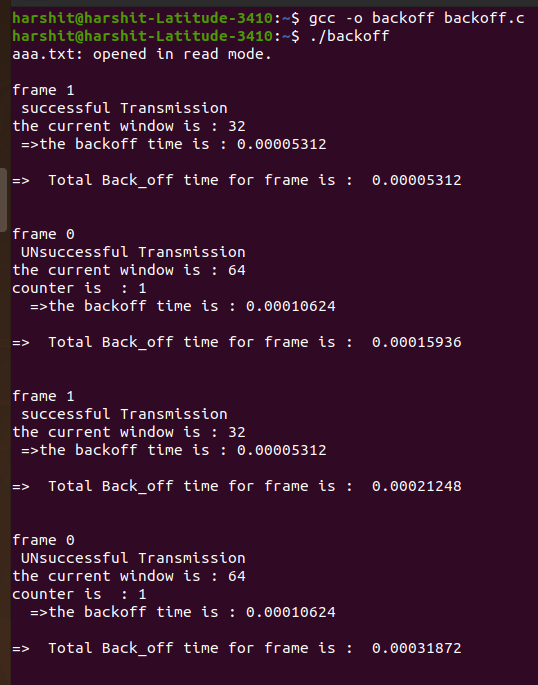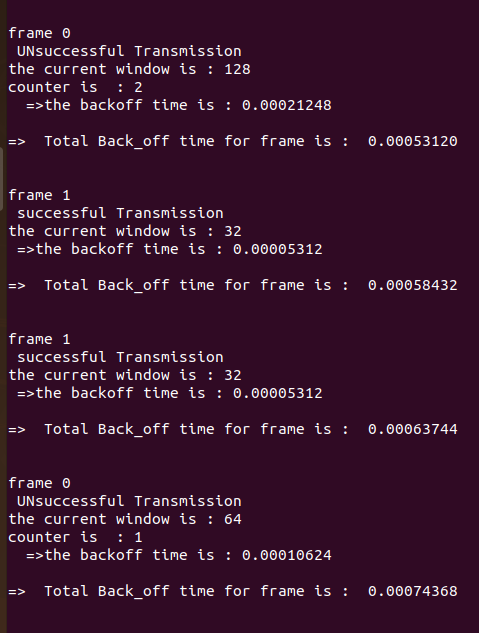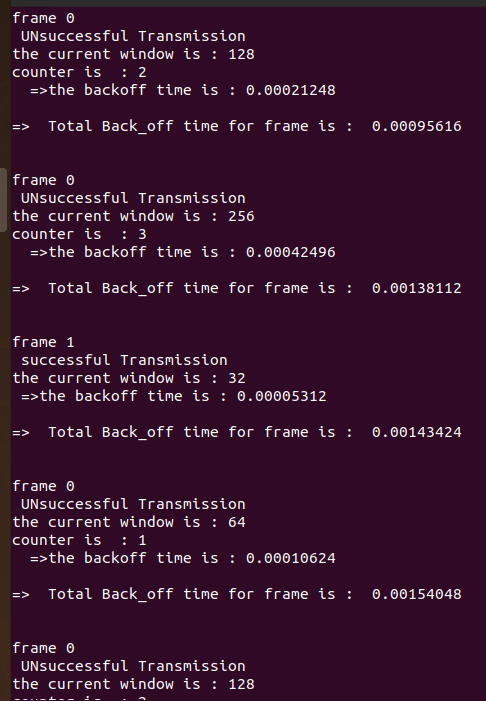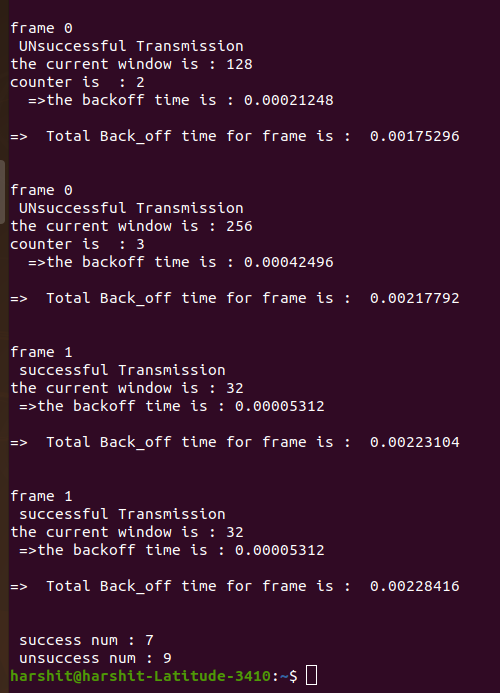Refined and Improved Binary Exponential Backoff Algorithm:

This algorithm (Also referred to as I-BEB in this article) aims to solve the fairness problem among nodes while transmitting up to the point and lowering the packet delay compare to BEB.

After the 12th time successful transmission, the counter is set to 1 and the contention window is exponentially increased. Still, I-BEB doesn’t solve the fairness problem much. Here in this algorithm, the threshold value is used because there can be a scenario where the number of the active station will increase and increase the chances of a collision. When CW is less than the threshold, it equals its contention window size to the threshold to increase the probability of successful transmission

C = 12, D = 4, E = 8, and G = 0.125 * CWmin
CWmin = 32 and CWmax = 1024

where,
C => Successful sending times
D => Quickly decrease the CW
E => Linearly increase the CW
G => Threshold quantity
CW => The minimum contention window size
BT => Backoff time

Below is the implementation of the above approach:

## C

 `// C program for the above approach`` ` `#include ``#include ``#include ``#include `` ` `// Driver Code``int` `main()`` ` `{``    ``int` `C = 12, D = 4, E = 8;`` ` `    ``int` `counter = 0;`` ` `    ``// Slot time``    ``double` `slot_time = 0.00000166,``           ``CW;``    ``int` `CWmax = 1024, CWmin = 32;``    ``double` `BT, G,``        ``Total_Backoff_time = 0;`` ` `    ``// C => successful sending times``    ``// D => Quickly decrease the the CW``    ``// E => linearly inc the CW``    ``// G => Threshold quantity``    ``// CW => Minimun Contention Window Size``    ``// BT => Backoff time`` ` `    ``// Enter the current BW``    ``printf``(``"Enter the Curr_BW (CW)"``           ``" by user  :  "``);``    ``scanf``(``"%lf"``, &CW);`` ` `    ``FILE``* fp;``    ``char``* f_name = ``"aaa.txt"``;``    ``char` `ch;`` ` `    ``// Open the file``    ``fp = ``fopen``(f_name, ``"r+"``);`` ` `    ``// If file doesn't exists``    ``if` `(fp == NULL) {``        ``printf``(``"%s does not exists"``               ``" \n"``,``               ``f_name);``        ``return` `0;``    ``}`` ` `    ``// Otherwise``    ``else` `{``        ``printf``(``"%s: opened in read"``               ``" mode.\n\n"``,``               ``f_name);``    ``}`` ` `    ``// Read character by characters``    ``while` `((ch = ``fgetc``(fp)) != EOF) {`` ` `        ``// End-of-file``        ``if` `(ch == ``'0'` `|| ch == ``'1'``) {``            ``printf``(``"frame %c \n "``, ch);``        ``}`` ` `        ``if` `(ch == ``'1'` `|| ch == ``'0'``) {`` ` `            ``if` `(counter < C) {`` ` `                ``// Print the counter``                ``// value``                ``counter = counter + 1;``                ``printf``(``"counter value"``                       ``" is : %d\n "``,``                       ``counter);`` ` `                ``CW = CW / D;``                ``printf``(``" The CW is :"``                       ``" %0.8f\n"``,``                       ``CW);`` ` `                ``G = 0.125 * CW;``                ``printf``(``"Value of G "``                       ``"[Threshold Quantity]"``                       ``" is : %0.8f\n"``,``                       ``G);`` ` `                ``if` `(CW < G) {``                    ``CW = G;``                    ``BT = CW * slot_time;``                    ``printf``(``                        ``" => The"``                        ``" Backoff Time"``                        ``" is : %0.8f\n"``,``                        ``BT);``                ``}``                ``else` `{``                    ``BT = CW * slot_time;``                    ``printf``(``                        ``" => The "``                        ``"Backoff Time"``                        ``" is : %0.8f\n"``,``                        ``BT);``                ``}``            ``}``            ``else` `{``                ``counter = 1;``                ``CW = CW + (E * CWmin);`` ` `                ``printf``(``" The CW is :"``                       ``" %lf\n"``,``                       ``CW);`` ` `                ``if` `(CW > CWmax) {``                    ``CW = CWmax;`` ` `                    ``BT = CW * slot_time;``                    ``printf``(``                        ``" => The "``                        ``"Backoff Time"``                        ``" is : %0.8f\n"``,``                        ``BT);``                ``}``                ``else` `{`` ` `                    ``BT = CW * slot_time;``                    ``printf``(``                        ``" => The "``                        ``"Backoff Time"``                        ``" is : %0.8f\n"``,``                        ``BT);``                ``}``            ``}``        ``}`` ` `        ``if` `(ch == ``'1'` `|| ch == ``'0'``) {`` ` `            ``// Find the Backoff time``            ``Total_Backoff_time = Total_Backoff_time + BT;`` ` `            ``printf``(``"\n"``);`` ` `            ``// Print the Back Off Time``            ``printf``(``"=>  Total Back_off"``                   ``" time for frame is : "``                   ``" %0.8f \n "``,``                   ``Total_Backoff_time);``            ``printf``(``"\n\n"``);``        ``}``    ``}``}`

Input File:

```1010011000100011
```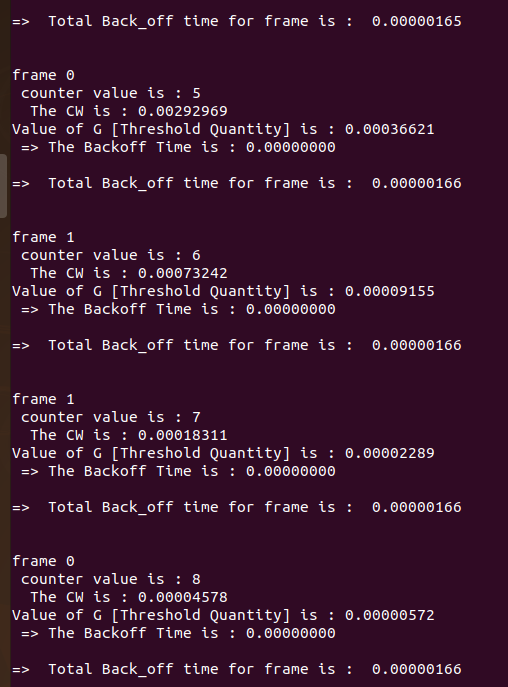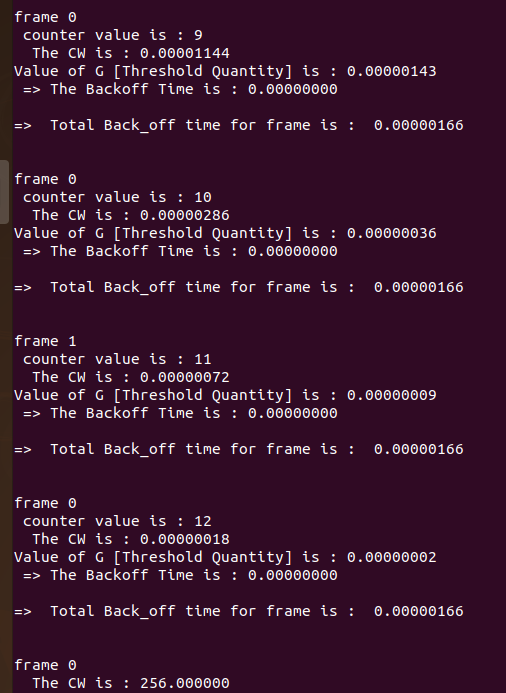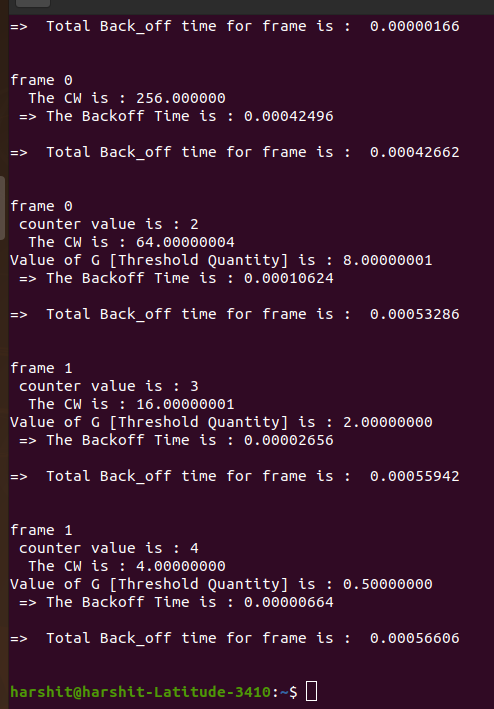Attention reader! Don’t stop learning now. Get hold of all the important CS Theory concepts for SDE interviews with the CS Theory Course at a student-friendly price and become industry ready.

My Personal Notes arrow_drop_up